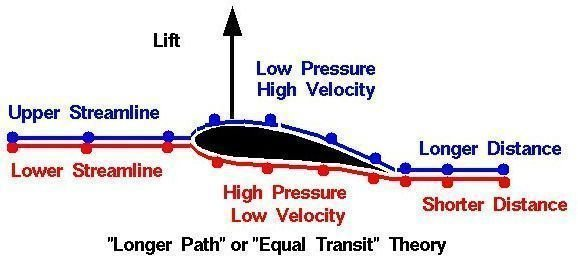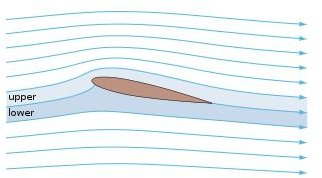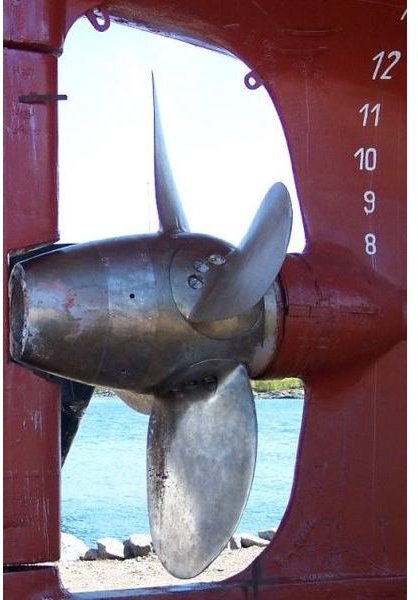# Euler’s Degrees of Vortex

## Introduction:

Euler’s degree of vortex comes under the dynamics of fluid. In this we study the velocity and acceleration at a point in a fluid flow, taking into consideration the forces causing the flow.

According to the Newton’s second law of motion, the net force Fx acting on a fluid element in the direction of x is equal to the mass of the fluid element multiplied by the acceleration ax in the x-direction.

Fx =m × ax.

Dynamics of fluid flow is the study of fluid motion with the forces causing the flow. The following forces are present in the fluid in motion.

• F g – Gravity Forces.
• F p – Pressure Forces.
• F v – Forces due to Viscosity.
• F t – Forces due to Turbulence.
• F c –Forces due to Compressibility.

The net forces acting on the fluid due to motion are:

F x = (F g) + (F p) + (F v) + (F t) + (F c).

1. From the above equation, if the (F c) is forces due to compressibility and is negligible the resulting net force is:

F x = (F g) + (F p) + (F v) + (F t).

And the equation is called the Reynolds’s Equation of Motion.

2. For the flow where (F t) forces due to turbulence is negligible the resulting equation of the motion are known as the Navier- Strokes Equation.

F x = (F g) + (F p) + (F v).

3. If the flow is assumed to be ideal viscous force (F v) is zero, the equation of motion is known as Euler’s equation of Motion.

F x = (F g) + (F p).

In the Euler’s equation of motion the forces due to the gravity and pressure are taken into consideration. This derived by considering the motion of a fluid element along a stream line flow.

Pressure force =(P + (∂p/∂s × ds)) × dA.

Pressure force in flow direction = P × dA.

Let Θ be the angle of flow .

The resultant flow on the fluid element in the direction of s must be equated to the mass of the fluid x acceleration in the direction of s.

CosΘ = dz/ds.

(∂p / p∂s)+(g × cosΘ) + (V× dv /∂s) =0

thus the Euler Equation of Motion :

(∂p/p) + (g× dz) + (V × dv ) = 0

## Euler’s Degrees of Vortex in Propeller Design:

Propeller Design:

• Hydrodynamic theory shows the flow an infinitely long circular cylinder in a non-viscous fluid. Here the velocity is zero and these are called stagnation points. The resultant force on the cylinder is zero.
• This flow can be transformed into flow around an aerofoil, with the stagnation points moving. The forces on the aerofoil in these conditions are also zero. It is because flow rounds the aerofoil without circulation.
• For flow around an aerofoil with circulation, the new pattern is the original pattern with the vortex superimposition upon it. The vortex is centered on the aerofoil, and the strength of its circulation depends upon the shape of the section and its angle of incidence. Its strength is such as to move the trailing edge. It can be shown that the lift on the aerofoil for given strength of calculation:

Lift = L = P × Vτ.

The fluid viscosity introduces a small drag force but has little influence on the lift generated.

The simple approach assumed an aerofoil of infinite span in which the flow would be two–dimensional. The lift force is generated by the difference in pressure on the face and back of the foil. In practice an aerofoil will be finite in span and there will be tendency for the pressure on the face and back to try to equalize at the tips by a flow around the ends of the span reducing the lift in these area.

## Hydrodynamic Theory of Aerofoil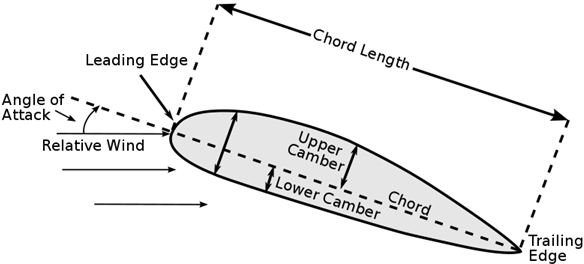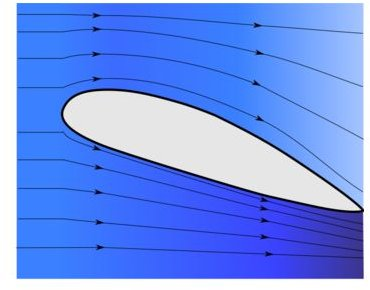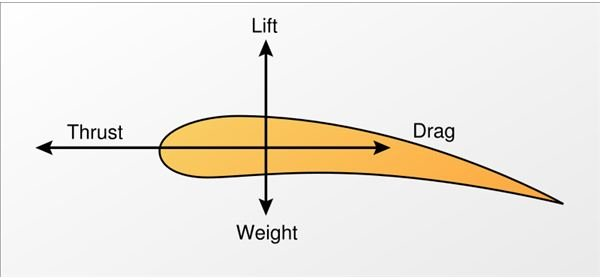## Pressure Distribution around the Aerofoil:

The effect of the flow past and circulation round the aerofoil is to increase the velocity over the back and reduce it over the face.By Bernoulli’s principle there will be a corresponding decrease in pressure over the back and an increase in pressure over the face.

Both the pressure distributions are contributing to the total lift, with the reduced pressure over the back making the greater contribution for the lift. The simple analysis ignores many factors which have to be taken into account in major comprehensive theories some of them are:

1. The finite number of blades and the variation in the axial and rotational in flow factor.
2. Interference effect between blades.
3. The flow around the tip from face to back of the blade which produce a tip vortex modifying the lift and drag for that region of the blade. It is not possible to cover adequately the more advance propeller theories in this article.

Some of the terminologies used in designing the propeller are:

• Lifting Line Model:

In these the aerofoil blade element is replaced with a single bound vortex at the radius concerned. The strength of the vortices varies with radius and line in the radial direction about which they act is called the lifting line.

• Lifting Surface Model:

In these the aerofoil is represented by an infinitely thin bound vortex sheet. The vortices in the sheet are adjusted to give the lifting characteristic of the blade.

• Surface Vorticity Model:

In this case rather than being arranged on a sheet, the vortices are arranged around the section.

• Vortex Lattice Model:

In such models the surface of the blade and its properties are represented by a system of vortex panels.

So these are some of the concepts based on the Euler’s degree of vortex is used in the designing of the propeller to create a maximum wake out of it and reduce the eddies formed by them.

Image Wikipedia – Airfoil

Image Wikipedia – Lift (Force)

Image Wikipedia – Controllable pitch propeller

## Pressure Distribution In Aerofoil